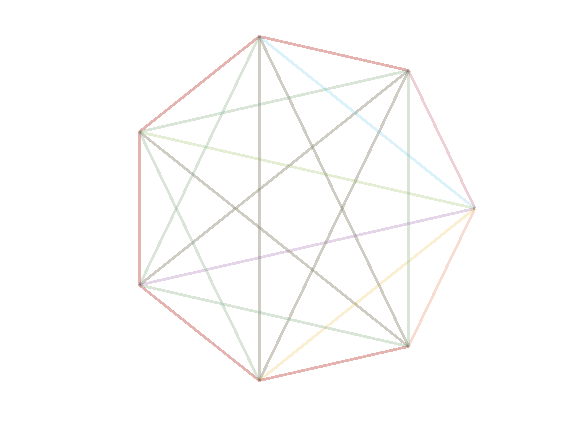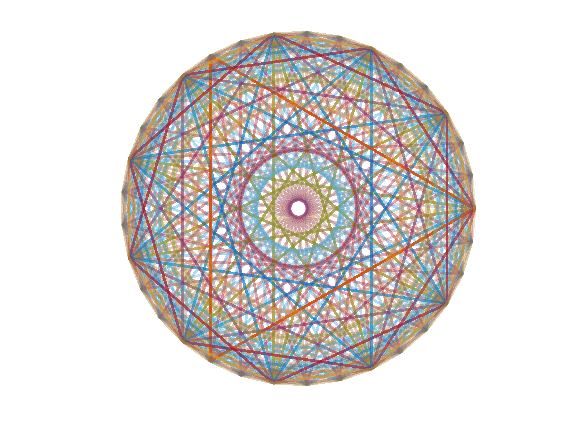# 🎧🎧🎧 Listen to Fourier transform 🎵🎵🎵Adam Danz on 28 Oct 2021
• 12
• 129
• 0
• 0
• 278
% 🎧🎧🎧 Listen to this one 🎵🎵🎵
% It produces an animation of 14 Fourier transform matricies
% The columns of each matrix produce a tone.
%
% https://blogs.mathworks.com/cleve/2014/09/29/finite-fourier-transform-matrix/
axis square off
hold on
% Cycle through matrix sizes defined in n
for n=7:2:33
% Set up data
x=fft(eye(n));
r=real(x);
m=imag(x);
% Set tone duration. Longer tones result is longer overlap.
d=.1; % seconds
% Replicate r values to duration length.
% Default sampling rate is 8192 hz.
s=8192*d; % ~number of samples needed to fill expected duration d.
a=repmat(r,ceil(s/n),1);
a(ceil(s):end,:)=[]; % trim excess samples
% h=gobjects(1,n); % Add this for cleaner code
cla
h(1)=plot(nan); % dummy line for first iteration
% Cycle through columns of matrix n
f=@(h)set(h,Color=[h.Color,.2],LineW=2);
for i=2:n
soundsc(a(:,i)) % play sound
f(h(i-1)) % change properties to previously drawn line
h(i)=plot(r(:,i),m(:,i),LineW=4); % plot(x(:,i)) won't work because some cols may not contain complex vals.
drawnow
end
f(h(i)) % change properties to last line
% pause(.1) % pause() is banned from the commpetition so we'll use a while-loop
tic
while toc<.1
end
end